# AP Physics 1 Question 325: Answer and Explanation

### Test Information

Question: 325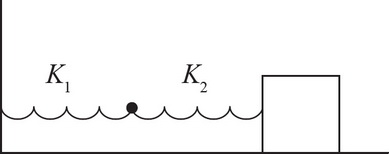3. The spring-block system above has a block of mass m oscillating in simple harmonic motion. However, instead of one spring, there are two springs connected to each other. The first spring has a spring constant k1, and the second has a spring constant k2. What would be the effective spring constant of the system above if k2 = 3k1 ?

• A. 4k1
• B. 3k1
• C. 4k1/3
• D. 3k1/4

The easiest way to solve this is to think qualitatively. A given force stretches each spring by a certain amount. When the springs are connected in series, the increases in length add together, but the tension forces don't. Thus, the object must move a larger distance to experience the same force from the springs. This is consistent with a reduced spring constant. Choice (D) is the only answer in which the new spring constant is less than the original, k1. If you want to see a proof, consider a situation in which the block is displaced some distance x from the equilibrium position. The first spring would be displaced some amount x1, and the second spring would be displaced some amount x2. Then you can say x = x1 + x2. Knowing that, F = -kx for all springs, you can say x1 = -F/k1 and x2 = -F/k2. Substituting those into the first equation gives x =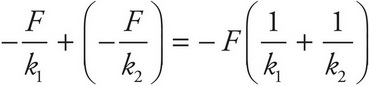. Solving for F then gives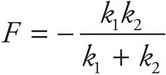. Therefore, the effective spring constant is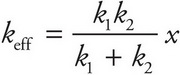. Plugging in the given values for this problem, you get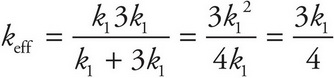.